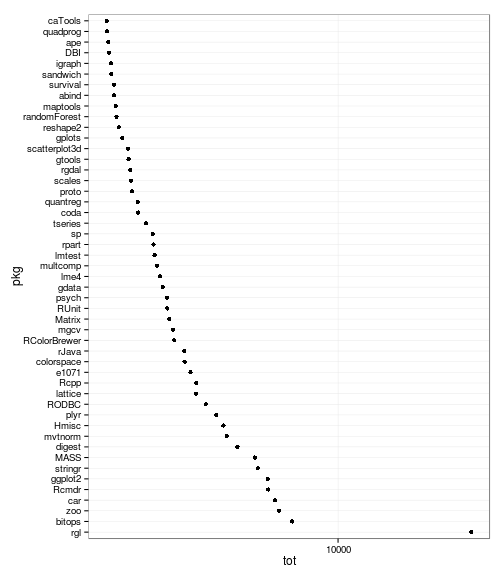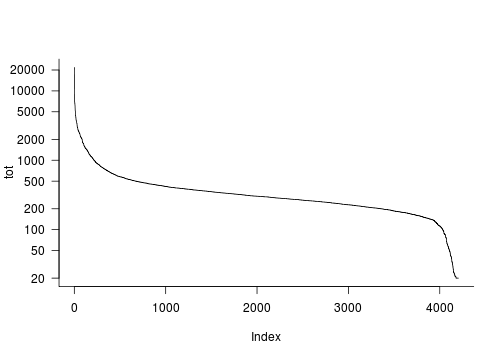# CRAN scraping for fun and ??profit ??

Version 2013-01-23 21:37:11

From time to time there are discussions on the R mailing lists and elsewhere about whether it would be possible for the R project to report on the relative popularity of R packages (e.g., this 2009 thread). This is typically met with a number of objections about the inaccuracy of such data: they would probably come from only a subset of CRAN mirrors (which might be unrepresentative of the global population); a package download is neither necessary nor sufficient to indicate that someone is actually using it; etc..

Jeff Ryan says in one such exchange:

While I think download statistics are potentially interesting for developers, done incorrectly it can very likely damage the community. A basic data reporting problem, with all of the caveats attached.

I appreciate the caution here, but I'm not exactly sure what the damage would be …

``````getBlob <- function(repos=getOption("repos"),blob=NULL) {
if (is.null(blob)) {
if (substr(repos,nchar(repos),nchar(repos))!="/")
repos <- paste0(repos,"/")
## does this work if getOption("repos") is @CRAN ?
## FIXME: check if this worked or not ... may not work for all repos
attr(blob,"repos") <- repos
}
return(blob)
}
scrapePackageStats <- function(...) {
require(stringr)
blob <- getBlob(...)
binstr <- c("/bin/.*/contrib/[^/]+/.*\\.tgz",  ## MacOS binaries
"/bin/.*/contrib/[^/]+/.*\\.zip",  ## Windows binaries
"/src/contrib/.*.tar.gz")          ## source
## pull all lines matching patterns
split1 <- unlist(lapply(binstr,grep,x=blob,value=TRUE))
## get package names
x2 <- str_extract(split1,pattern="[^/]+\\.(tgz|tar\\.gz|zip)")
x3 <- as.numeric(gsub("^.*class=\"R\">([0-9]+).*\$","\\1",split1))
## could also distinguish by OS version ...
x4 <- tapply(x3,list(x2),sum)
d <- data.frame(pkgfull=names(x4),n=x4)
rownames(d) <- NULL
transform(d,
pkg=gsub("_.*\$","",pkgfull),
num=gsub(".*_([0-9.-]+)\\..*\$","\\1",pkgfull),
type=str_extract(pkgfull,"(tar\\.gz|tgz|zip)\$"))
}
``````

``````(L <- load("CRANblob.RData"))
``````
``````##  "blob"
``````
``````d2 <- scrapePackageStats(blob=blob)
``````
``````library(plyr)
d4 <- ddply(d2,"pkg",summarise,tot=sum(n))  ## aggregate to sums
d5 <- transform(d4,
pkg=factor(pkg,levels=as.character(pkg)[rev(order(tot))]),
rank=rank(-tot,ties.method="first"))
``````
``````library(ggplot2)
theme_set(theme_bw())
qplot(tot,pkg,data=subset(d5,as.numeric(pkg)<50))+scale_x_log10()
``````Some packages of particular interest:

``````subset(d5,pkg %in% c("emdbook","mixstock","bbmle","lme4","phylobase"))
``````
``````##            pkg  tot rank
## 219      bbmle  643  419
## 972    emdbook  487  711
## 1892      lme4 3542   25
## 2168  mixstock  389 1201
## 2678 phylobase  455  829
``````

``````with(d5[order(d5\$rank),],plot(tot,log="y",type="l"))
``````Read `packages.rds` file (based at least in part on this StackOverflow question)

``````recent.packages.rds <- function() {
mytemp <- tempfile()
mytemp)
stringsAsFactors=FALSE)
mydata[["Published"]] <- as.Date(mydata[["Published"]])
#sort and get the fields you like:
mydata
}
``````
``````rpkg <- recent.packages.rds()
``````
``````stripwhite <- function(s) {
gsub("^[[:space:]]+","",
gsub("[[:space:]]+\$","",s))
}
rpkg2 <- transform(subset(rpkg,select=c(Package,Author)),
Author=stripwhite(gsub("^([^,<&;\\[]+).*","\\1",
gsub("^(.+)(and|by|AND|with|Contributions).*",
"\\1",Author))))
## View(table(rpkg2\$Author))
``````

(Trying to extract just first author names. Formatting of authors is very messy … would maintainers be easier??)

## To do

• merge CRAN info on package maintainers etc.? (e.g. `all Hadley packages'',`R-core packages'', etc.); number of reverse-depends/suggests/enhances; etc.
• is r-forge scrapeable in a similar way?
• other sources: CRANberries; Google scholar citations; ??

The mailing list threads linked above points to a web site at UCLA that posts these sorts of stats; however, it seems quite out of date (web info says last modified Sep 2010; lists 2.11.1 as the most popular version of R; and a haphazard sample of the packages listed shows that they are not current versions).